# Example Question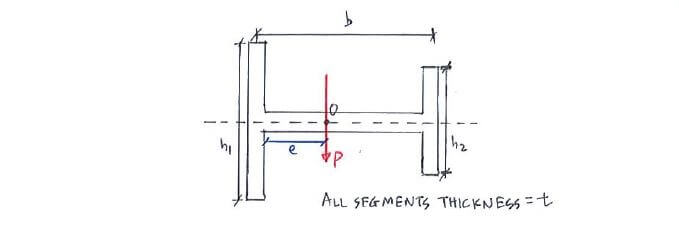Click below to show answer. X

#### Step 1: Identify forces required for ΣM = Pe Step 1: Identify forces required for ΣM = Pe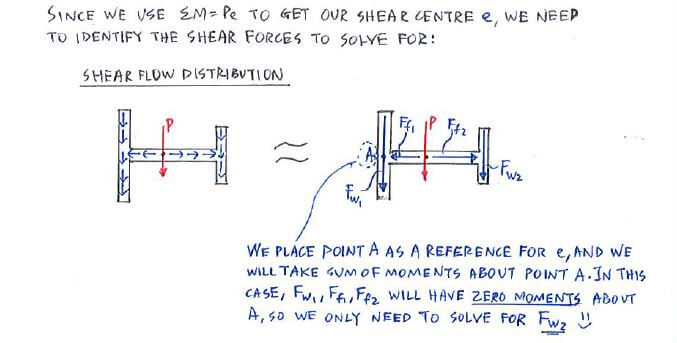#### Step 4: Use V = ∫q ds to calculate Fw2 Step 4: Use V = ∫q ds to calculate Fw2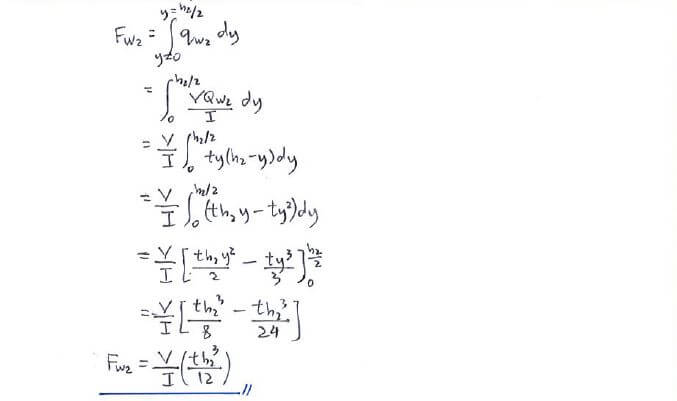#### Step 5: Use ΣMA = Pe to find shear centre e Step 5: Use ΣMA = Pe to find shear centre e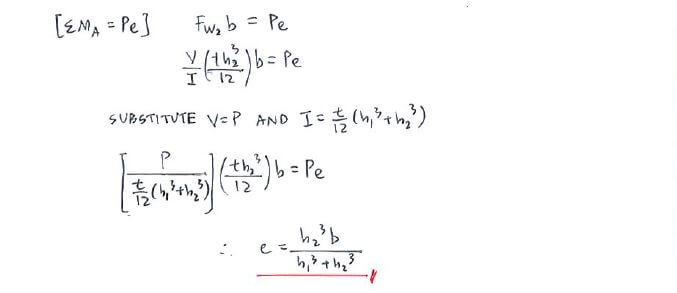#### Useful formula: Useful formula: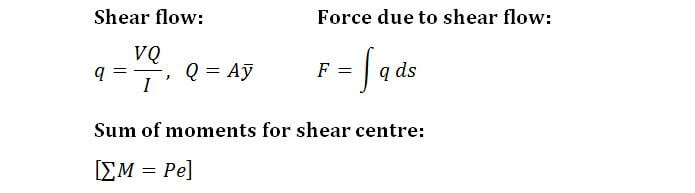Now that you've mastered this question, you can try Question 1.